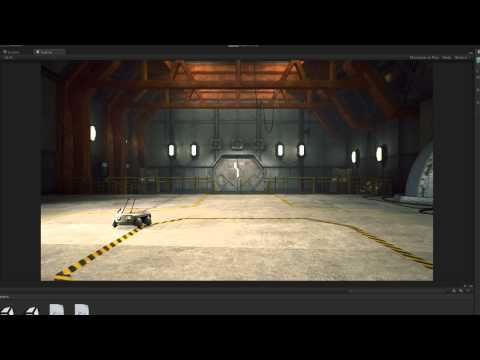# コルーチン

#### 難易度: 中級## コルーチン

### Code snippet

``````using UnityEngine;
using System.Collections;

public class CoroutinesExample : MonoBehaviour
{
public float smoothing = 1f;
public Transform target;

void Start ()
{
StartCoroutine(MyCoroutine(target));
}

IEnumerator MyCoroutine (Transform target)
{
while(Vector3.Distance(transform.position, target.position) > 0.05f)
{
transform.position = Vector3.Lerp(transform.position, target.position, smoothing * Time.deltaTime);

yield return null;
}

print("Reached the target.");

yield return new WaitForSeconds(3f);

print("MyCoroutine is now finished.");
}
}``````
``````#pragma strict

public var smoothing : float = 1f;
public var target : Transform;

function Start ()
{
MyCoroutine(target);
}

function MyCoroutine (target : Transform)
{
while(Vector3.Distance(transform.position, target.position) > 0.05f)
{
transform.position = Vector3.Lerp(transform.position, target.position, smoothing * Time.deltaTime);

yield;
}

print("Reached the target.");

yield WaitForSeconds(3f);

print("MyCoroutine is now finished.");
}``````
``````import UnityEngine
import System.Collections

public class CoroutinesExample(MonoBehaviour):

public smoothing as single = 1.0F

public target as Transform

private def Start():
StartCoroutine(MyCoroutine(target))

private def MyCoroutine(target as Transform) as IEnumerator:
while Vector3.Distance(transform.position, target.position) > 0.05000000075F:
transform.position = Vector3.Lerp(transform.position, target.position, (smoothing * Time.deltaTime))

yield null

print('Reached the target.')

yield WaitForSeconds(3.0F)

print('MyCoroutine is now finished.')``````

### Code snippet

``````using UnityEngine;
using System.Collections;

public class PropertiesAndCoroutines : MonoBehaviour
{
public float smoothing = 7f;
public Vector3 Target
{
get { return target; }
set
{
target = value;

StopCoroutine("Movement");
StartCoroutine("Movement", target);
}
}

private Vector3 target;

IEnumerator Movement (Vector3 target)
{
while(Vector3.Distance(transform.position, target) > 0.05f)
{
transform.position = Vector3.Lerp(transform.position, target, smoothing * Time.deltaTime);

yield return null;
}
}
}``````
``````#pragma strict

public var smoothing : float = 7f;
private var target : Vector3;

function  SetTarget(value : Vector3)
{
target = value;

StopCoroutine("Movement");
StartCoroutine("Movement", target);
}

function Movement (target : Vector3)
{
while(Vector3.Distance(transform.position, target) > 0.05f)
{
transform.position = Vector3.Lerp(transform.position, target, smoothing * Time.deltaTime);

yield;
}
}``````
``````import UnityEngine
import System.Collections

public class PropertiesAndCoroutines(MonoBehaviour):

public smoothing as single = 7.0F

public Target as Vector3:
get:
return target
set:
target = value

StopCoroutine('Movement')
StartCoroutine('Movement', target)

private target as Vector3

private def Movement(target as Vector3) as IEnumerator:
while Vector3.Distance(transform.position, target) > 0.05000000075F:
transform.position = Vector3.Lerp(transform.position, target, (smoothing * Time.deltaTime))

yield null``````

### Code snippet

``````using UnityEngine;
using System.Collections;

public class ClickSetPosition : MonoBehaviour
{
public PropertiesAndCoroutines coroutineScript;

void OnMouseDown ()
{
Ray ray = Camera.main.ScreenPointToRay(Input.mousePosition);
RaycastHit hit;

Physics.Raycast(ray, out hit);

if(hit.collider.gameObject == gameObject)
{
Vector3 newTarget = hit.point + new Vector3(0, 0.5f, 0);
coroutineScript.Target = newTarget;
}
}
}``````
``````#pragma strict

public var coroutineScript : PropertiesAndCoroutines;

function OnMouseDown ()
{
var ray : Ray = Camera.main.ScreenPointToRay(Input.mousePosition);
var hit : RaycastHit;

Physics.Raycast(ray, hit);

if(hit.collider.gameObject == gameObject)
{
var newTarget : Vector3 = hit.point;
coroutineScript.SetTarget(newTarget);
}
}``````
``````import UnityEngine
import System.Collections

public class ClickSetPosition(MonoBehaviour):

public coroutineScript as PropertiesAndCoroutines

private def OnMouseDown():
ray as Ray = Camera.main.ScreenPointToRay(Input.mousePosition)
hit as RaycastHit

Physics.Raycast(ray, hit)

if hit.collider.gameObject == gameObject:
newTarget as Vector3 = (hit.point + Vector3(0, 0.5F, 0))
coroutineScript.Target = newTarget``````